• 向量是由n个实数组成的一个n行1列（n*1）或一个1行n列（1*n）的有序数组； 向量的点乘,也叫向量的内积、数量积，对两个向量执行点乘运算，就是对这两个向量对应位一一相乘之后求和的操作，点乘...对于向量a和向量b

向量是由n个实数组成的一个n行1列（n*1）或一个1行n列（1*n）的有序数组；

向量的点乘,也叫向量的内积、数量积，对两个向量执行点乘运算，就是对这两个向量对应位一一相乘之后求和的操作，点乘的结果是一个标量。

点乘公式

对于向量a和向量b：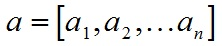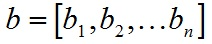a和b的点积公式为：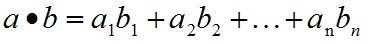要求一维向量a和向量b的行列数相同。

点乘几何意义

点乘的几何意义是可以用来表征或计算两个向量之间的夹角，以及在b向量在a向量方向上的投影，有公式：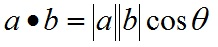推导过程如下，首先看一下向量组成：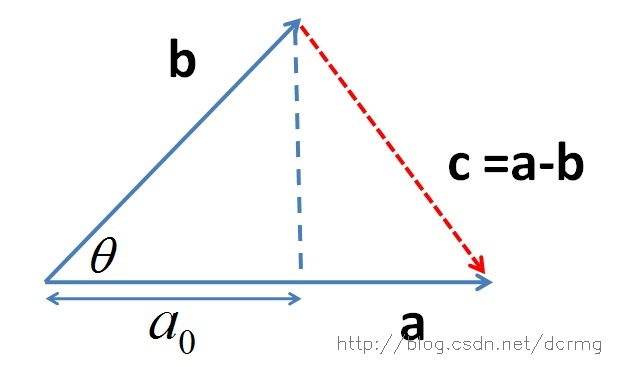定义向量：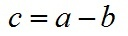根据三角形余弦定理有：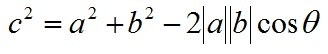根据关系c=a-b（a、b、c均为向量）有：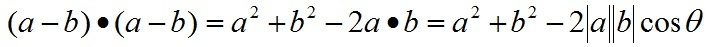即：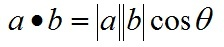向量a，b的长度都是可以计算的已知量，从而有a和b间的夹角θ：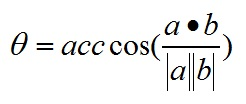根据这个公式就可以计算向量a和向量b之间的夹角。从而就可以进一步判断这两个向量是否是同一方向，是否正交(也就是垂直)等方向关系，具体对应关系为：

a·b>0    方向基本相同，夹角在0°到90°之间
a·b=0    正交，相互垂直
a·b<0    方向基本相反，夹角在90°到180°之间

叉乘公式

两个向量的叉乘，又叫向量积、外积、叉积，叉乘的运算结果是一个向量而不是一个标量。并且两个向量的叉积与这两个向量组成的坐标平面垂直。

对于向量a和向量b：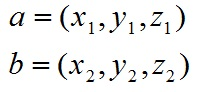a和b的叉乘公式为：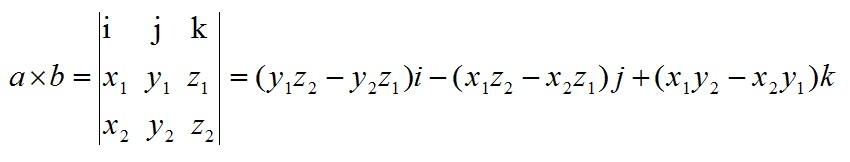其中：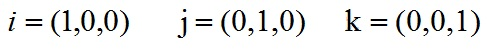根据i、j、k间关系，有：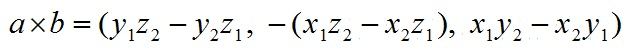叉乘几何意义

在三维几何中，向量a和向量b的叉乘结果是一个向量，更为熟知的叫法是法向量，该向量垂直于a和b向量构成的平面。

在3D图像学中，叉乘的概念非常有用，可以通过两个向量的叉乘，生成第三个垂直于a，b的法向量，从而构建X、Y、Z坐标系。如下图所示：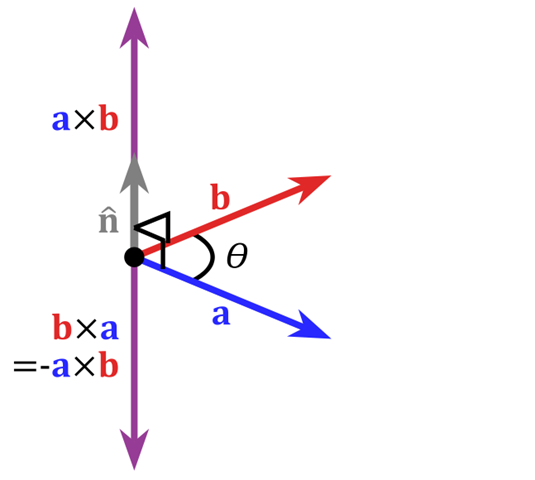在二维空间中，叉乘还有另外一个几何意义就是：aXb等于由向量a和向量b构成的平行四边形的面积。


展开全文• 要求一维向量a和向量b的行列数相同。 2、内积的几何意义 点乘的几何意义是可以用来表征或计算两个向量之间的夹角，以及在b向量在a向量方向上的投影。 二、向量的外积和几何意义（叉乘） 两个向量的外积，又叫...
目录：

一、向量的内积和几何意义

二、向量的外积和几何意义

一、向量的内积和几何意义（点乘）

对于向量a和向量b：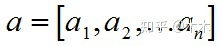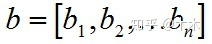1、a和b的内积公式为：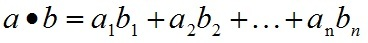要求一维向量a和向量b的行列数相同。

2、内积的几何意义

点乘的几何意义是可以用来表征或计算两个向量之间的夹角，以及在b向量在a向量方向上的投影。

二、向量的外积和几何意义（叉乘）

两个向量的外积，又叫向量积、叉乘等。外积的运算结果是一个向量而不是一个标量。并且两个向量的叉积与这两个向量组成的坐标平面垂直。

对于向量a和向量b：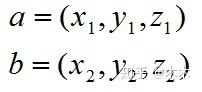1、a和b的外积公式为：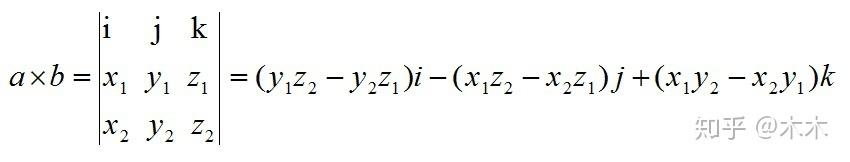其中：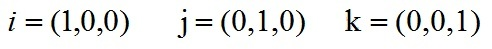根据i、j、k间关系，有：2、叉乘几何意义

在三维几何中，向量a和向量b的叉乘结果是一个向量，更为熟知的叫法是法向量，该向量垂直于a和b向量构成的平面。

展开全文• 要求一维向量a和向量b的行列数相同。点乘的结果是一个标量而不是向量 定义：两个向量a与b的内积为 a·b = |a||b|cos∠(a, b)，0·a =a·0 = 0；若a，b是非零向量，则a与b向量正交的充要条件是a·b = 0。 向量内积的...
向量
已知a、b、c是三个向量
向量的投影  投影过程
$a向量在b向量上的投影就是作a到b的垂线，交点就是投影坐标$
向量的长度（向量的模）
$向量a 的长度 记作 |a|$$|a| = \sqrt{a.x^2 + a.y^2 + a.z^2}$
向量的单位
1向量单位 = 向量长度为1的向量
也就是1 = |a|
向量归一化
$向量归一化 =\frac {向量的分量} {所有向量的分量的和}$$normal(a(x,y,z)) =( \frac{a.x}{a.x + a.y+a.z}, \frac{a.y}{a.x + a.y+a.z}, \frac{a.z}{a.x + a.y+a.z})$
求与原点、a、b构成的平行四边形点c
$c = a + b$
求直线AB的中点C
$c =( a + b ) / 2$
求向量AB的方向(0，c)
$c = a - b$
求向量a延长N倍后的向量c
$向量 * 常数 = 延长向量 ，c = aN = a.xN, a.yN$
向量的内积（点乘）
向量的内积记作a·b ，结果是个常数
$【公式】$ $a·b= a.x \times b.x + a.y \times b.y= |a||b|cos∠(a, b)$
$【计算过程】$$根据向量的减法得到：c = a-b$$根据余弦定理得到：c^2 = a^2 + b^2 -2ab$$展开后得到：(a-b)(a-b)=a^2 + b^2 -2|a||b|cos∠(a,b)$$简化后得到：a·b = |a||b|cos∠(a, b)$
计算a、b两个向量之间的夹角
$a·b = |a||b|cos∠(a,b)$
$∠(a,b) = arccos(a·b|a||b|), (内积变体)$
判断a、b两个向量的方向
$a dot b =$
$公式：n =a·b = |a||b|cos∠(a, b)$$n>0，说明夹角在0°到90°之间，方向基本相同$$n=0，说明相互垂直$$n<0，说明夹角在90°到180°之间，两个向量方基本相反$
$【用途3：b向量在a向量方向上的投影】$向量的投影是指：作b到a的垂线，垂线交点就是b向量在a向量上的投影$b在a的投影：n=\frac{a·b}{|a|}$
向量的外积（叉乘）
向量a与b的外积记作a×b，结果是一个向量
该向量的其长度等于原点向量a与向量b组成的平行四边形的面积=|a×b| = |a||b|sin∠(a,b)，
【公式与过程】
$a \times b =\begin{vmatrix} i & j & k \\ a.x & a.y & a.z \\ b.x & b.y&b.z \end{vmatrix};\begin{pmatrix}i、j、k为代表向量参数位置的向量 \\ i=(1,0,0); j=(0,1,0) ;k=(0,0,1) \end{pmatrix}$$=(a.y*b.z - b.y*a.z) i-(a.x*b.z - b.x *a.z) j+(a.x*b.y - b.x *a.y) k$$=((a.y*b.z - b.y*a.z),0,0)-(0,(a.x*b.z - b.x *a.z),0)+(0,0,(a.x*b.y - b.x *a.y))$$=((a.y∗b.z−b.y∗a.z), -(a.x∗b.z−b.x∗a.z), (a.x∗b.y−b.x∗a.y))$
【性质】
$a × b = -b × a. （反称性）;(λa + μb) × c = λ(a ×c) + μ(b ×c). （线性）$
在二维世界中，求向量a和向量b构成的平行四边形的面积
$c = |a \times b|$
求垂直于a，b向量的法向量c，从而构建X、Y、Z坐标系
$其方向正交于a与b。并且，(a,b,a×b)构成右手系。$$c=a\times b$


展开全文• 向量内积的理解向量内积的定义向量内积的性质向量内积的几何意义向量内积可以做...这里要求一维向量a和向量b的行列数相同。注意：点乘的结果是一个标量(数量而不是向量) 定义：两个向量a与b的内积为 a·b = |a||b...
向量内积的理解向量内积的定义向量内积的性质向量内积的几何意义向量内积可以做什么
向量内积的定义
概括地说，向量的内积（点乘/数量积）。对两个向量执行点乘运算，就是对这两个向量对应位一一相乘之后求和的操作，如下所示，对于向量a和向量b：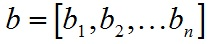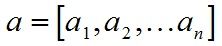a和b的点积公式为：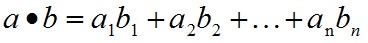这里要求一维向量a和向量b的行列数相同。注意：点乘的结果是一个标量(数量而不是向量)
定义：两个向量a与b的内积为 a·b = |a||b|cos∠(a, b)，特别地，0·a =a·0 = 0；若a，b是非零向量，则a与b为正交的充要条件是a·b = 0。
向量内积的性质

a^2 ≥ 0；当a^2 = 0时，必有a = 0. （正定性）
a·b = b·a. （对称性）
(λa + μb)·c = λa·c + μb·c，对任意实数λ, μ成立. （线性）
cos∠(a,b) =a·b/(|a||b|).
|a·b| ≤ |a||b|，等号只在a与b共线时成立.

向量内积的几何意义
内积（点乘）的几何意义包括：

表征或计算两个向量之间的夹角
b向量在a向量方向上的投影
公式：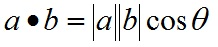向量内积可以做什么
一个重要的应用就是可以根据内积判断向量a和向量b之间的夹角和方向关系,具体来说：
a·b>0    方向基本相同，夹角在0°到90°之间
a·b=0    正交，相互垂直
a·b<0    方向基本相反，夹角在90°到180°之间
简单来说就是内积可以反映出两个向量之间的某种关系或联系。


展开全文• 内积 计算公式 几何意义 ...向量b向量a投影 推导过程 外积 计算公式 几何意义 两个向量构成平面法向量 构件三维坐标系 外积在数值上等于两个向量组成平行四边形面积 reference ...
•基本概念
• 参考： https://blog.csdn.net/dcrmg/article/details/52416832 ... 1 向量内积（点乘） ...a和b的点积（点乘）公式为： 向量内积的几何意义及用途 包括： 表征或计算两个向量之间的夹角 b向量在a向量...
• 点乘、数量积、内积 向量点乘即数量积,记作a·b；其中a·b=｜a｜·｜b｜cosθ,｜a｜、｜b｜是两向量模,θ是两向量之间夹角(0≤θ≤π)....|向量c|=|向量a×向量b|=|a||b|sin<a,b> 向量c方向与a
• 要求一维向量a和向量b的行列数相同 点乘的几何意义是可以用来表征或计算两个向量之间的夹角，以及在b向量在a向量方向上的投影 根据这个公式就可以计算向量a和向量b之间的夹角。从而就可以进一步判断这两个向量是否是...
• 声明： 本文转自这里 向量是由n个实数组成的一个n行1列（n*1）或一个1行n列（1*n）的有序数组； 向量的点乘,也叫向量的内积、数量积，对...对于向量a和向量b： &nbsp; &nbsp; &nbsp; &...点乘
• 向量的内积（点乘） ...这里要求一维向量a和向量b的行列数相同。注意：点乘的结果是一个标量(数量而不是向量) 定义：两个向量a与b的内积为 a·b = |a||b|cos∠(a, b)，特别地，0·a =a·0 = 0...
• 点乘,也叫向量的内积、数量积.顾名思义,求下来的结果是一个数. 向量a·向量b=|a||b|cos 在物理学中,已知力与位移求功,实际上就是求向量F与向量s的内积,即要用点乘. 向量叉乘：a ∧ b（常被写为a x b） 叉乘,也叫...
• 已知两个非零向量a、b，那么|a||b|cosθ（θ是a与b的夹角）叫做a与b的数量积或内积。记作a·b。两个向量的数量积等于它们对应坐标的乘积的。即：若a=(x1,y1),b=(x2,y2)，则a·b=x1·x2+y1·y2 设a、b为非零向量，...
• ## 向量内积和基变换

千次阅读 2018-08-23 10:48:33
向量B的模为1，则AB的内积值等于A向B所在直线投影的矢量长度 其实就是将B看成是一个基。 比如说原来在（0，1），（1，0）这个基下，（2，3）。 要求在这一组基的坐标，就可以直接内积 写成矩阵的形式就是： ...降维 线性变换...